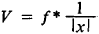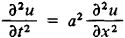# Generalized Functions

The following article is from The Great Soviet Encyclopedia (1979). It might be outdated or ideologically biased.

## Generalized Functions

also distributions, a mathematical concept that generalizes the classical concept of function. The need for such generalization arises in many physical and mathematical problems. On the one hand, the concept of generalized function makes it possible to express in mathematically correct form such idealized concepts as the (spatial) density of a point mass, the density of a surface charge layer or dipole layer, the intensity of an instantaneous source, and so on. On the other hand, the concept of generalized function reflects the fact that the value of a physical quantity at a point cannot actually be measured; one can only average it over sufficiently small neighborhoods of the point. Thus, generalized functions serve as a convenient tool for describing the distributions of various physical quantities. Therefore, generalized functions are also called distributions.

Generalized functions were introduced at the end of the 1920’s by P. Dirac in his studies on quantum mechanics, in which he systematically made use of the concept of the delta function and its derivatives. The foundations of the mathematical theory of generalized functions were laid by S. L. Sobolev in 1936 in his solution of the Cauchy problem for hyperbolic equations. In the postwar period, the French mathematician L. Schwartz provided a systematic exposition of the theory of generalized functions. The theory was subsequently intensively developed by many mathematicians, primarily to meet the requirements of mathematical physics. The theory of generalized functions has numerous applications and is being increasingly used by physicists, mathematicians, and engineers.

Generalized functions are formally defined as continuous linear functionals on a given linear space of test functions ø(x). An example of such a space is the set of infinitely differentiable finite functions furnished with an appropriate motion of convergence (more accurately, an appropriate topology). In this case, ordinary locally summable functions f(x) are identified with functionals (regular generalized functions) of the type

(1) (f, ø) = ∫ f(x)ø(x) dx

The derivative of a generalized function f is a functional f’ defined by the equation

(f’, ø) = (f, ø’)

Thus, a generalized function is infinitely differentiable in the generalized sense. By virtue of (1), equation (2) is just a generalization of the formula for integration by parts of functions f(x) differentiable in the ordinary sense, so that for such functions both concepts of derivative coincide.

Convergence in a (linear) set of generalized functions is defined as weak convergence of functionals. It turns out that the operation of differentiation of generalized functions is continuous, and a convergent sequence of generalized functions allows term-by-term differentiation an infinite number of times.

Other operations on generalized functions, such as the convolution of functions, the Fourier transform, and the Laplace transform, are also introduced. The theory of these operations assumes its simplest and most complete form within the framework of the concept of generalized function, which expands the potential of classical mathematical analysis. The use of generalized functions therefore significantly expands the range of problems that can be considered and leads to significant simplifications by “automating” elementary operations.

Examples. (1) The Dirac delta function, (δ, ø) = ø (0), describes the density of a unit mass (or charge) concentrated at the point x = 0, or a unit impulse.

(2) θ(x) is the Heaviside function. θ(x) = 0, for x ≤ 0, and θ(x) = 1, for x > 0; θ’ = δ, that is, its derivative describes a unit impulse.

(3) –δ’ describes a unit dipole at the point x = 0, oriented along the x-axis.

(4) μδs describes α density of a simple layer on a surface S with surface density μ:

(μδs, ø) = sμ(x)ø(x) dS

(5) –∂/∂n(νδs) describes a dipole-layer distribution of density ν on a surface S. The dipole moment of the layer is oriented along the normal n to the surface:(6) The convolutionis the Newtonian potential associated with density f where f is any generalized function [for example, of (1), (3), (4), and (5)].

(7) The general solution of the equation of a vibrating string,is given by the formula

u(x, t) = f(x + at) + g(x - at)

where f and g are any generalized function.

### REFERENCES

Dirac, P. A. M. Osnovy kvantovoi mekhaniki. Moscow-Leningrad, 1932. (Translated from English.)
Soboleff, S. “Méthode nouvelle à resoudre le problème de Cauchy pour les équations linéaires hyperboliques normales.” Matematicheskii sbornik, 1936, vol. 1 (43), no. 1. (Abstract in Russian.)
Schwartz, L. Théorie des distributions, vols. 1–2. Paris, 1950–51.
Gel’fand, I. M., and G. E. Shilov. Obobshchennye funktsii i deistviia nad nimi, 2nd ed. Moscow, 1959.
Vladimirov, V. S. Uravneniia matematicheskoi fiziki, 2nd ed. Moscow, 1971.

V. S. VLADIMIROV

The Great Soviet Encyclopedia, 3rd Edition (1970-1979). © 2010 The Gale Group, Inc. All rights reserved.
References in periodicals archive ?
Kratzel, "Integral transformations of Bessel-type," in Generalized functions and operational calculus (Proc.
A possibility in studying stochastic differential equations is to make use of the theory of Colombeau-type generalized functions spaces to overcome the multiplication problem in distribution space.
Some generalized functions for the size distribution of income.
A new approximate method to calculate the critical forces for the singular type elastic foundation plates is suggested; this method departing from the approximation of corresponding differential equations' coefficients using generalized functions. The elaborated method can serve is basis for researching the loss of stability of real engineering structures having elastic foundation and elastic supports.
Finally, we have obtained the columns of magnetic and electricmatrixGreen's function in the space of generalized functions (distributions) and their regularizations (approximations).
Reif (1982, 1985a) differentiated scales into five different functions: abrasion resistance, defense, drag reduction, bioluminescence and generalized functions, and indentified eight characteristic morphologies associated with these functional types.
Shapes of generalized functions which describe experimental points for criterion [k.sub.1]
We claim that the tanh method can be extended by replacing tanh function with some generalized functions f(x), such as polynomial function, trigonometric function and Jacobi elliptical function.
We also generalize these functions and gave the functional equations satisfied by these generalized functions and then show that they satisfy Ramanujan's description of mock theta functions.
Distributions; generalized functions with applications in Sobolev spaces.
This is a consequence of a forthcoming impossibility theorem, stating that enforcing a prolongation of the convolution product from a subset of distributions (for which the commutative and associative convolution product is defined) to a superset of generalized functions, while retaining all product properties, makes us loose the 1 ideal [N'.sub.0].

Site: Follow: Share:
Open / Close